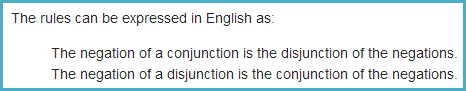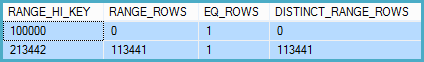Including my content from SQLBlog.com and some from SQLPerformance.com

## Tuesday 15 April 2014

### Cardinality Estimation for Disjunctive (OR) Predicates in SQL Server 2014 Onward

Cardinality Estimation for Disjunctive Predicates in SQL Server 2014

## Introduction

Back in January 2014, I wrote an article called Cardinality Estimation for Multiple Predicates that described the cardinality estimation process for queries with multiple predicates, from the point of view of the old and new cardinality estimators.

The article describes the various behaviours and formulas involved, along with the usual sprinkling of documented and undocumented trace flags. I described the formula SQL Server 2014 uses to calculate a cardinality estimate for multiple predicates connected with `AND` (conjunctive predicates), which was already relatively well-known.

Despite some fairly energetic research, and basic-to-intermediate skills with Excel, I was unable to deduce a similar formula for the disjunctive case, where predicates are connected by `OR`. The trace flag output I describe in the other article clearly showed that exponential backoff was used in the new 2014 cardinality estimator, but the precise formula eluded me.

I gave up on it until a few weeks ago, when I was invited to contribute to the technical review of a White Paper Joe Sack was writing. One of the contributions I was able to make was to point out that exponential backoff was used for disjunctions as well as conjunctions.

The paper has now been released so I can now write about the detail behind the statement that appears there concerning cardinality estimation for disjunctive predicates (thank you Joe for pointing this out to me):### A Bit Of Boolean Logic

The key bit of new information is the second sentence. As soon as I read it, a vague memory from my early education came back to me, as well as from a cunning query rewrite Itzik Ben-Gan shows in one of his books. Both relate to a set of rules for transforming Boolean algebra, known as DeMorgan’s Laws. Wikipedia has this to say:This gives us a general way to turn a disjunction into a conjunction.

It is a short step from there to understand that exponential backoff for disjunctions in the SQL Server 2014 cardinality estimator works by applying DeMorgan’s laws.

Converting the troublesome `OR` to an `AND` (with the appropriate negations) allows us to reuse the `AND` exponential backoff calculation for `OR` predicates.

### The Theory

Borrowing from the Wikipedia article again, we have DeMorgan’s Laws stated as:The interesting transformation from disjunction to conjunction can be expressed as:

`not (A or B)` is the same as `(not A) and (not B)`

This does not quite give us a direct translation from `OR` to `AND`. We need the extra step of observing:

`(A or B)` is the same as `not (not (A or B))`

This trivial double-negative means we can now directly substitute the `not (A or B)` above, to get:

`(A or B)` is the same as `not ((not A) and (not B))`

This gives us `OR` expressed in terms of `NOT` and `AND`.

The last bit of theory we need is that A and B are probabilities expressed as a fraction between 0 and 1, so:

`(not X) = (1 – X)`

## Worked Example

Now we have all we need to show how the disjunctive exponential backoff calculation works in SQL Server 2014 using the AdventureWorks sample database:

``````SELECT
NumRows = COUNT_BIG(*)
FROM Production.TransactionHistory AS TH
WHERE
TH.TransactionID BETWEEN 100000 AND 168412
OR TH.TransactionDate BETWEEN '20070901' AND '20080313';
``````

There are two predicates in this query, connected by `OR.`

The selectivity of the predicate on `Transaction ID` can be derived directly from the statistics histogram:

``````DBCC SHOW_STATISTICS
(
'Production.TransactionHistory',
'PK_TransactionHistory_TransactionID'
)
WITH HISTOGRAM;
``````

The output is:This very compact histogram gives us all the information we need to say that the range of 68,413 `Transaction ID` values can be expected to produce 68,413 rows.

Expressed as a fraction of the 113,443 rows in the table, the selectivity of this predicate is 68413 / 113443 = 0.603061. We will call this value selectivity `A`.

The second predicate (on `TransactionDate`) is estimated from the histogram in a similar way (except the histogram has more steps than is convenient to show here).

The histogram calculation results in a cardinality estimate for this predicate that is also 68,413 (because I deliberately chose the predicates to qualify exactly the same physical rows).

The selectivity is likewise 0.603061. We will call this value selectivity `B`.

### Enter DeMorgan

We know from our earlier work with DeMorgan that:

`(A or B)` is the same as `not ((not A) and (not B))`

So we can rewrite our `(A or B)` predicate immediately as `not ((not A) and (not B))`. This means we can stop worrying about the `OR`.

Note that this transformation is done purely for cardinality estimation purposes — the `OR` is not removed from the optimizer tree.

### Doing the Math

Now, given that selectivities `A` and `B` are both equal to 0.603061 in this contrived example, our expanded expression becomes:

``````not ((not 0.603061) and (not 0.603061))
``````

We also know:

`(not X) = (1 – X)`

Expanding the inner `not`s means the computation becomes:

``````not ((1 - 0.603061) and (1 - 0.603061))
``````

Now we can perform the numeric math inside the parentheses to get:

``````not (0.396939 and 0.396939)
``````

Now we use exponential backoff to compute the `AND` selectivity:

``````not (0.396939 * SQRT(0.396939))
``````

And replace the outer `not` just as we did before:

``````1 - (0.396939 * SQRT(0.396939))
``````

Now we have just numbers. The final selectivity is 0.749916.

## The Result

The final step is to multiply this selectivity by the cardinality of the base table to get our overall estimate:

`0.749916 * 113443 = 85073` (rounded up)

The 2014 execution plan for this query using the new cardinality estimator is:The cardinality estimate is 85,073 rows, just as we predicted.

The actual number of rows returned by this query is 68,413 rows (because both predicates qualify exactly the same rows). Nothing is perfect, and cardinality estimation doubly so.